Miscellaneous

Chapter 7 Class 12 Integrals
Serial order wise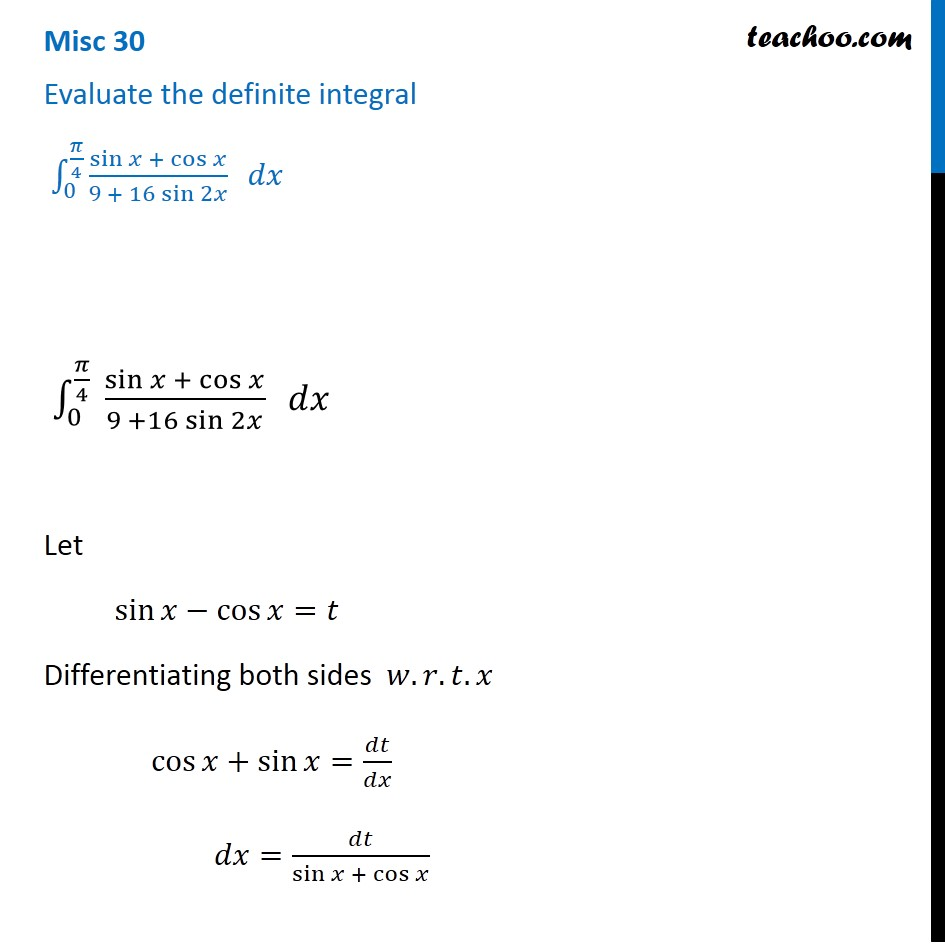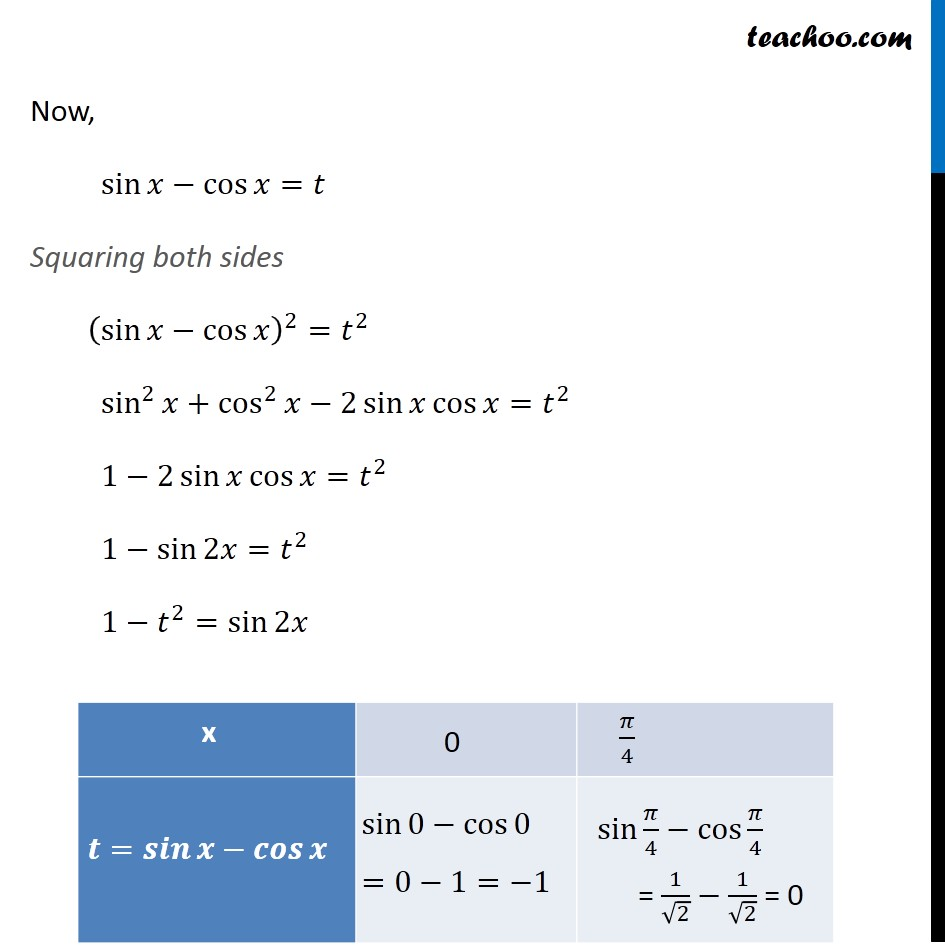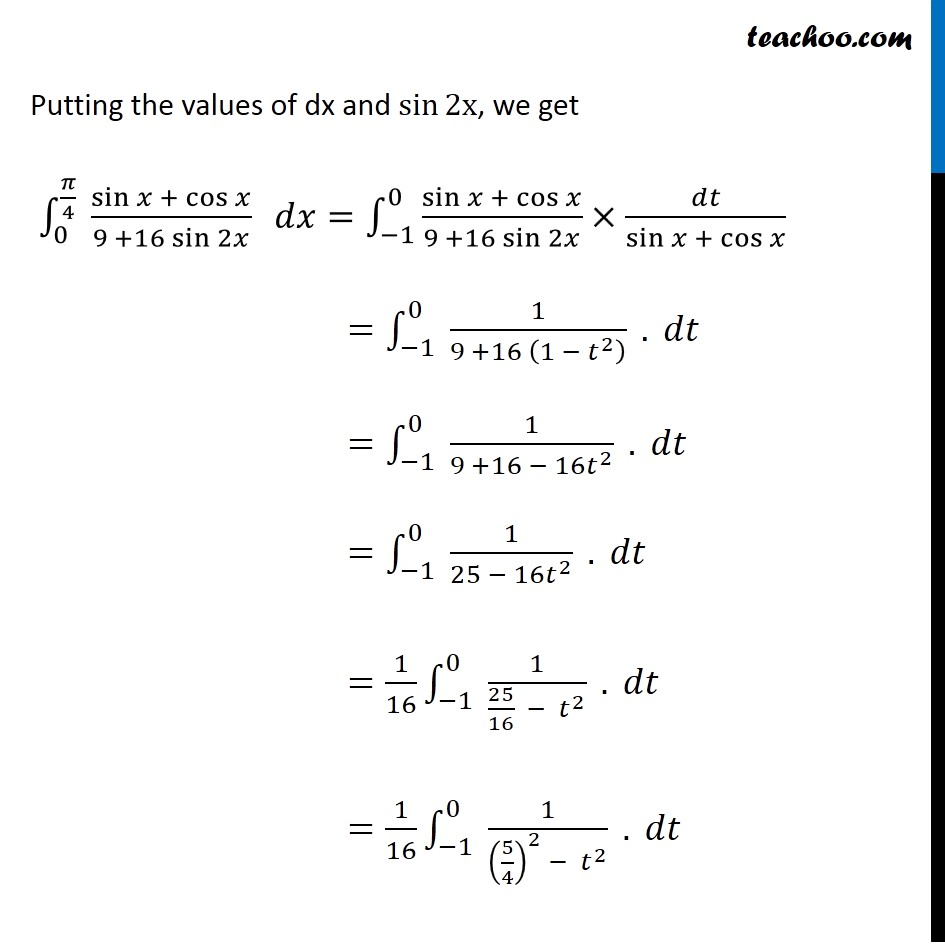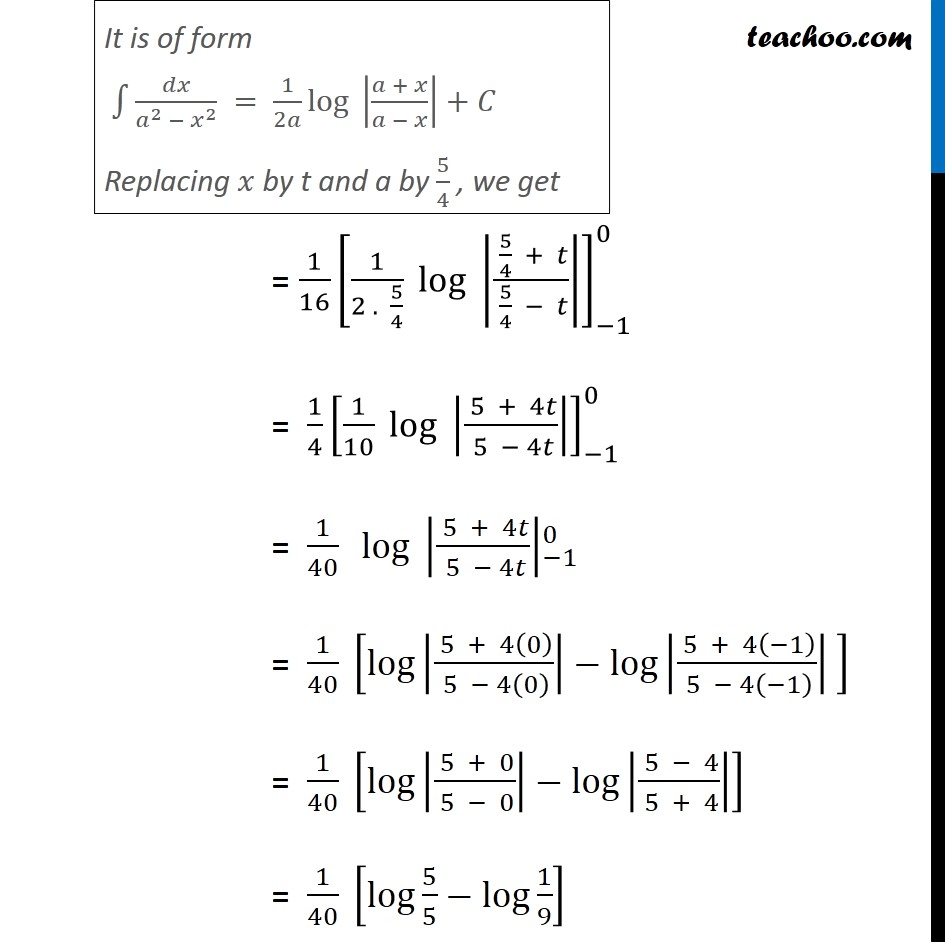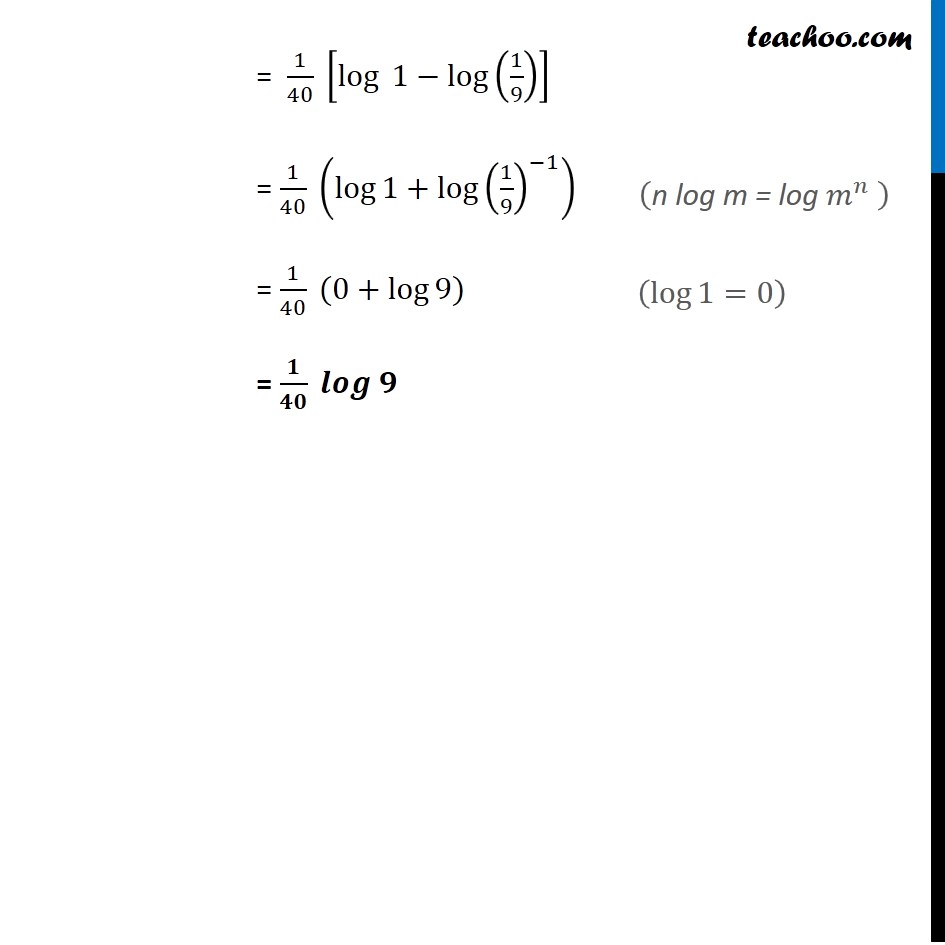Solve all your doubts with Teachoo Black (new monthly pack available now!)

### Transcript

Misc 30 Evaluate the definite integral ∫_0^(𝜋/4)▒〖(sin⁡𝑥 + cos⁡𝑥)/(9 + 16 sin⁡2𝑥 ) 〗 𝑑𝑥 ∫_0^(𝜋/4)▒〖 (sin⁡𝑥 + cos⁡𝑥)/(9 +16 sin⁡2𝑥 ) 〗 𝑑𝑥 Let sin⁡𝑥−cos⁡𝑥=𝑡 Differentiating both sides 𝑤.𝑟.𝑡.𝑥 cos⁡𝑥+sin⁡𝑥=𝑑𝑡/𝑑𝑥 𝑑𝑥=𝑑𝑡/(sin⁡𝑥 + cos⁡𝑥 ) Now, sin⁡𝑥−cos⁡𝑥=𝑡 Squaring both sides (sin⁡𝑥−cos⁡𝑥 )^2=𝑡^2 sin^2⁡𝑥+cos^2⁡𝑥−2 sin⁡𝑥 cos⁡𝑥=𝑡^2 1−2 sin⁡𝑥 cos⁡𝑥=𝑡^2 1−sin⁡2𝑥=𝑡^2 1−𝑡^2=sin⁡2𝑥 sin⁡0−cos⁡0 =0−1=−1 Putting the values of dx and sin⁡2x, we get ∫_0^(𝜋/4)▒〖 (sin⁡𝑥 + cos⁡𝑥)/(9 +16 sin⁡2𝑥 ) 〗 𝑑𝑥=∫1_(−1)^0▒〖(sin⁡𝑥 + cos⁡𝑥)/(9 +16 sin⁡2𝑥 )×𝑑𝑡/(sin⁡𝑥 + cos⁡𝑥 )〗 =∫_(−1)^0▒〖 1/(9 +16 (1 − 𝑡^2 ) ) 〗. 𝑑𝑡 =∫_(−1)^0▒〖 1/(9 +16 − 16𝑡^2 ) 〗. 𝑑𝑡 =∫_(−1)^0▒〖 1/(25 − 16𝑡^2 ) 〗. 𝑑𝑡 =1/16 ∫_(−1)^0▒〖 1/(25/16 − 𝑡^2 ) 〗. 𝑑𝑡 =1/16 ∫_(−1)^0▒〖 1/((5/4)^2 − 𝑡^2 ) 〗. 𝑑𝑡 It is of form ∫▒〖𝑑𝑥/(𝑎^2 − 𝑥^2 ) = 1/2𝑎 〖log 〗⁡|(𝑎 + 𝑥)/(𝑎 − 𝑥)|+𝐶〗 Replacing 𝑥 by t and a by 5/4 , we get = 〖1/16 [1/(2 . 5/4) 〖 log〗⁡〖 |( 5/4 + 𝑡)/( 5/4 − 𝑡)|〗 ]〗_(−1)^0 = 〖1/4 [1/10 〖 log〗⁡〖 |( 5 + 4𝑡)/( 5 − 4𝑡)|〗 ]〗_(−1)^0 = 1/40 〖log⁡〖 |( 5 + 4𝑡)/( 5 − 4𝑡)|〗〗_(−1)^0 = 1/40 [log⁡|( 5 + 4(0))/( 5 − 4(0) )|−log⁡|( 5 + 4(−1))/( 5 − 4(−1) )| ] = 1/40 [log⁡|( 5 + 0)/( 5 − 0)|−log⁡|( 5 − 4)/( 5 + 4)| ] = 1/40 [log⁡〖5/5〗−log⁡〖1/9〗 ] = 1/40 [〖log 〗⁡1−log⁡(1/9) ] = 1/40 (log⁡1+log⁡〖(1/9)^(−1) 〗 ) = 1/40 (0+log⁡〖9)〗 = 𝟏/𝟒𝟎 𝒍𝒐𝒈 𝟗 ("n log m = log " 𝑚^𝑛 " " ) (log⁡〖1=0〗 )